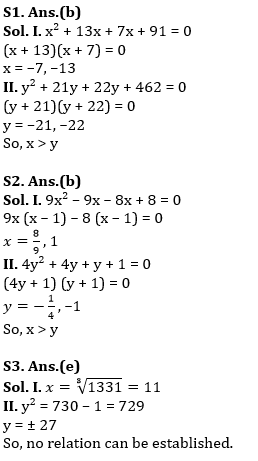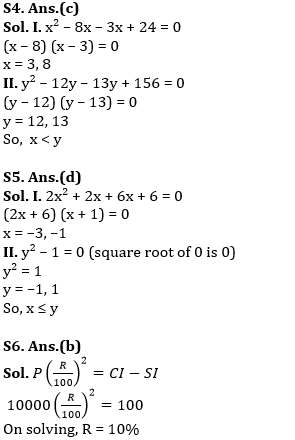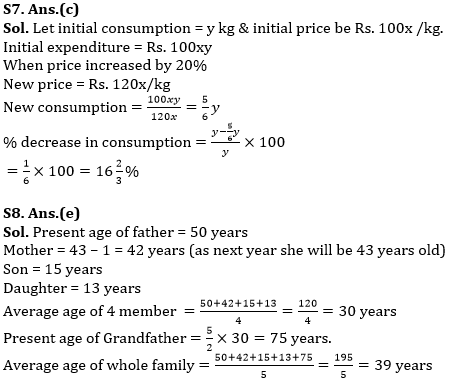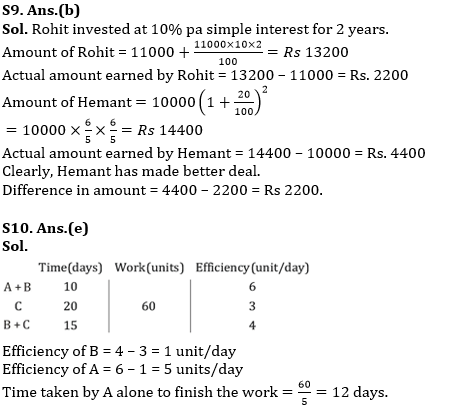Latest Banking jobs   »

# Quantitative Aptitude Quiz For RBI Assistant/ ESIC UDC Mains 2022- 3rd April

Directions (1-5): Solve the given quadratic equations and mark the correct option based on the answer.
(a)x ≥ y
(b) x > y
(c) x < y
(d) x ≤ y
(e) x = y or no relation can be established

Q1. I. x² + 20x + 91 = 0
II. y² + 43y + 462 = 0

Q2. I. 9x² – 17x + 8 = 0
II. 4y² + 5y + 1 = 0

Q3. I. x³ = 1331
II. y² + 1 = 730

Q4. I. x² – 11x + 24 = 0
II. y² – 25y + 156 = 0

Q5. I. 2x² + 8x + 6 = 0
II. (y² – 1)² = 0

Q6. If the difference of compound interest and simple interest on a sum of Rs. 10000 after 2 years is Rs.100, then find the rate of interest if interest is compounded annually. (in % p.a.)
(a) 9%
(b) 10%
(c) 11%
(d) 15%
(e) 20%

Q7. With the increase in price of sugar by 20%, a family has to decrease their consumption to keep expenditure same. Find percentage decrease in consumption.
(a) 20%
(b) 25%
(c) 16⅔%
(d) 33⅓%
(e) 10%

Q8. The present age of Father is 50 years while Mother will turn 43 years next year. The age of their son and daughter is 15 years and 13 years respectively. Also,the present age of Grandfather is 5/2 times of the average age of Father, mother, son and daughter. What is the average age of the family (all 5 members)?
(a) 30 years
(b) 30 years 3 months
(c) 39 years 3 months
(d) 40 years
(e) 39 years

Q9. Rohit & Hemant won a cash award of Rs. 11000 & Rs. 10000 respectively in a race competition. Both decided to invest their respective amount in two schemes. Rohit invested in scheme A offering 10% p.a. interest (simple) while Hemant invested in scheme B offering 20% rate of interest per annum compounding annually. Who has made better deal and by how much if both invested for 2 years?
(a) Rohit, Rs. 1200
(b) Hemant, Rs. 2200
(c) Both has made equal deal
(e) Rohit, Rs. 1100

Q10. A work can be finished by A & B in 10 days when they work together while C alone can finish the same work in 20 days. If B and C working together can complete the same work in 15 days, then in how many days can A alone finish the same work?
(a) 6⅔ days
(b) 20 days
(c) 7 ½ days
(d) 15 days
(e) 12 days

Solutions